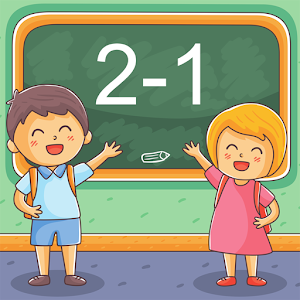Nederlands

We bieden dagelijks GRATIS gelicenseerde Android apps en games aan, die je normaal zou moeten kopen.\$2.99
VERSTREKEN

# Android Giveaway of the Day - First grade Math - Subtraction

Practice and improve math skills.
\$2.99 VERSTREKEN
Waarderingscijfer: 0 (0%) 0 (0%)

Deze giveaway is verlopen. First grade Math - Subtraction is nu beschikbaar op de normale manier.

Intuitive interface powered by handwriting input and a fun and engaging mini game in addition to a regular math trainer mode make our app stand out from the crowd of generic math learning apps.

With First grade Math - Subtraction you can practice and improve the following math skills:
- Subtraction facts - numbers up to 10
- Subtraction facts - numbers up to 18
- Subtraction facts - numbers up to 20
- Subtract multiples of 10
- Subtract a multiple of ten
- Subtract one-digit number from two-digit number
- Subtract two two-digit numbers

Sergey Malugin

Education

### Versie:

Varies with device

### Grootte:

Varies with device

Everyone

### Compatibiliteit:

Varies with device

## Reactie op First grade Math - Subtraction

Thank you for voting!